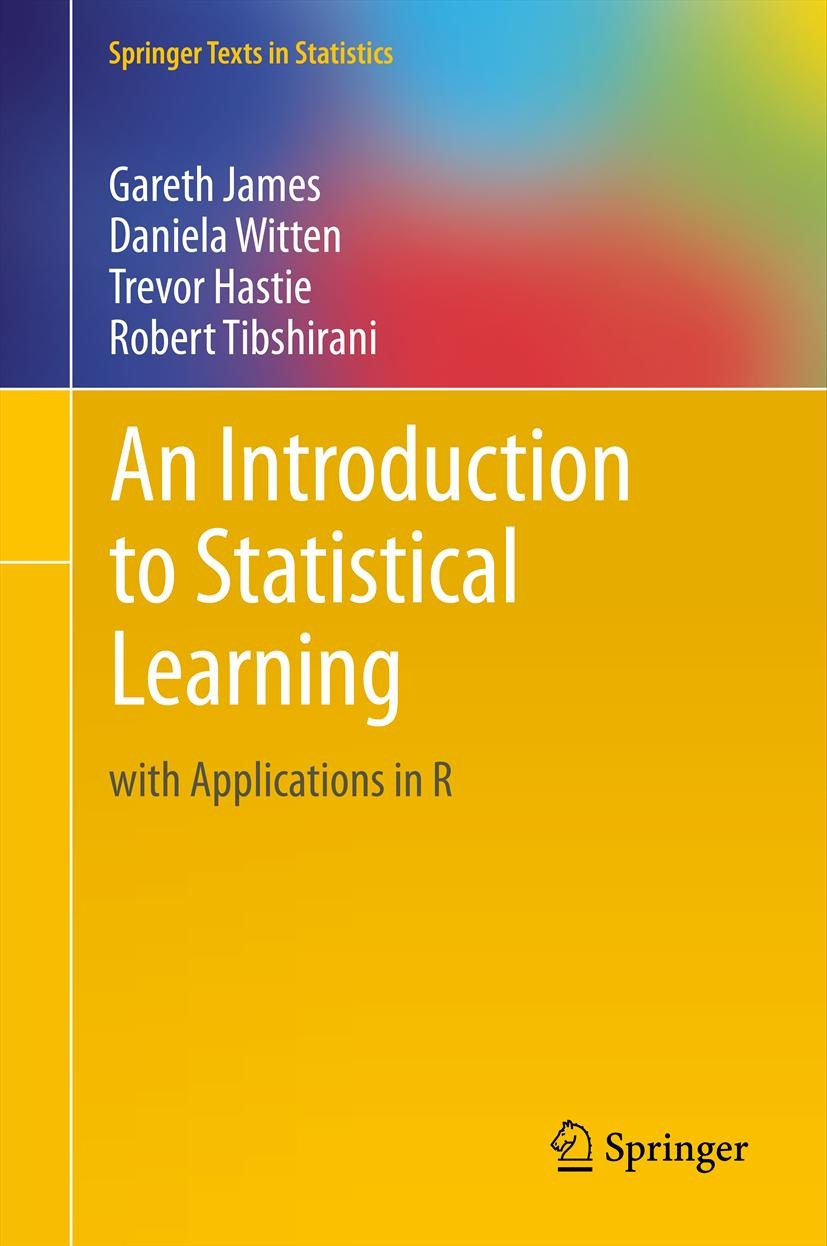An Introduction to Statistical Learning

with Applications in R

 HomeAbout this Book R Code for Labs Data Sets and Figures ISLR Package Get the Book Author Bios Errata

Errata for the 1st Edition, since the 7th printing (June 2017) and not reflected in online version

Page 254. The glmnet package has been updated so two lines of code need to change. The line

ridge.pred=predict(ridge.mod,s=0,newx=x[test,],exact=T) should be changed to

ridge.pred=predict(ridge.mod,s=0,newx=x[test,],exact=T,x=x[train,],y=y[train])

predict(ridge.mod,s=0,exact=T,type="coefficients")[1:20,] should be changed to

predict(ridge.mod,s=0,exact=T,type="coefficients",x=x[train,],y=y[train])[1:20,]

The code on the website has been updated accordingly.

Thanks to

Lenna Choi

Errata for the 1st Edition, prior to the 7th printing (June 2017) and reflected in online version

Page 52. exercise 2(c), should read "interested in predicting"

Page 72. The p-value for the Newspaper coefficient in Table 3.3 should be 0.00115.

Page 211. After “…with each response measurement in (6.1).” Add “Typically \hat \sigma^2 is estimated using the full model containing all predictors.”

Page 212. Equation 6.3 should have a \hat \sigma^2 in the denominator with n, in the same way as for the equation above it for AIC.

Page 232. The principal components in Figure 6.16 were calculated after first standardizing both pop and ad, a common approach. Hence, the x-axes on Figures 6.15 and 6.16 are not on the same scale.

Page 347. Lines 5 and 15. The references to (9.14) are incorrect. The reference should be to (9.15).

Thanks to

Poul Navin, David Dalpiaz, Jiao Wang, Hiroshi Ochiumi, Jayanth Raman

Errata for the 1st Edition, prior to the 6th printing (October 2015) and reflected in online version

Page 24, at the end of the last line it should read Fig 2.4 instead of Fig 2.3.

Page 66, line 16: The line starting “This estimate is known as the residual standard error …” should read “The estimate of $\sigma$ is known as the residual standard error …”

Page 96, the caption to Figure 3.11 should read "Right: The response has been log transformed…".

Page 104, In Question 7 the reference to Section 3.3.3 should be to 3.3.2.

Page 144, Should read "For these data we don't expect this to be a problem, since p=2 and n=10,000," since p=2 not 3 or 4.

Page 150, the first line after Figure 4.9 should be as follows "is some multiple of 1,275" (not 1,225).

Page 334, Q9(b), should read “… Purchase as the response and the other variables as predictors….”

Page 342, the caption for Figure 9.3 should read "The two blue points and the purple point that lie on the dashed lines are the support vectors, and the distance from those points to the hyperplane is indicated by arrows." Currently the text says margin rather than hyperplane.

Page 364, The “newx=dat[-train,]” in the R command at the bottom of the page is incorrect. It should read “newdata=dat[-train,]”. Correspondingly the 39% at the bottom of the page should be 10%. The R code on the website contains the corrected command.

Page 415, The text for exercise 6(b) should read "The researcher decides to replace the (j,i)th element of X with x_{ji} - \phi_{j1} z_{i1} where..." An additional like should read "(The principal component analysis is performed on X^T)."

Thanks to

Ilya Kavalerov, Vladimir Vovk, Gongkai Li, Marisano James, Paulo Orenstein, Bob Stine, Thomas Lengauer and Scott Kaiser.

Errata for the 1st Edition, prior to the 4th printing (June 2014) and reflected in online version

Page 149, (4.23) is missing the -1/2 log|\Sigma_k| term.

Page 211: The equation Cp=sigma hat^2(Cp'+n) should be Cp=sigma hat^2/n(Cp'+n)

Page 217, scale invariant should be scale equivariant.

Page 254: The parameter to control the number of folds in cv.glmnet() is "nfolds" not "folds".

Page 260, Exercise 3 and 4: the sum of square terms should be squared.

Page 295: “The generic plot() function recognizes that gam2 is an object of class gam," should read “The generic plot() function recognizes that gam.m3 is an object of class gam,”

Page 318, figure 8.8: the colors for OOB: Bagging and OOB: RandomForest are interchanged in the legend. OOB: RandomForest is lowest.

Page 333, exercise 8 should refer to test MSE rather than test error rate.

Thanks to

Iain Pardoe, Vladimir Vovk, Wei Jiang, Oscar Bonilla, Mette Langaas, Antoine Freches,  Graeme Blackwood, and Adele Cutler.